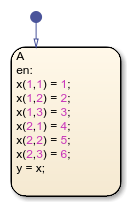## Select Array Layout for Matrices in Generated Code

When generating code from a Stateflow® chart that uses C as the action language, you can specify the array layout for matrices. For example, consider this matrix:By default, the code generator uses column-major layout to flatten the matrix as a one-dimensional array. The array is stored in memory with this arrangement:

`{1, 4, 2, 5, 3, 6}`
If you select row-major layout, the code generator flattens the matrix and stores it in memory with this arrangement:
`{1, 2, 3, 4, 5, 6}`
If you have Embedded Coder®, you can preserve the multidimensionality of Stateflow local data, prevent its flattening, and implement the matrix as a two-dimensional array with this arrangement:
`{{1, 2, 3}, {4, 5, 6}}`
Row-major and multidimensional array layout can ease the integration with external code. For more information, see Code Generation of Matrices and Arrays (Simulink Coder) and Dimension Preservation of Multidimensional Arrays (Embedded Coder).

### Column-Major Array Layout

By default, the Array Layout configuration parameter for a Simulink® model is `Column-Major`. When you press Ctrl-B, the code generator flattens all matrix data into one-dimensional arrays, arranging their contents in a column-major layout.

For example, this Stateflow chart contains local data `x` of size `[2 3]`. The state actions index the elements in `x` by row and column number.If you generate code, the file `sf_matrix_layout.c` implements the local data `x` in column-major layout with these lines of code:

```... sf_matrix_layout_DW.x = 1.0; sf_matrix_layout_DW.x = 2.0; sf_matrix_layout_DW.x = 3.0; sf_matrix_layout_DW.x = 4.0; sf_matrix_layout_DW.x = 5.0; sf_matrix_layout_DW.x = 6.0; ...```

The generated code refers to the elements of `x` by using only one index.

### Row-Major Array Layout

Row-major layout can improve the performance of certain algorithms. For example, see Interpolation Algorithm for Row-Major Array Layout (Embedded Coder).

To generate code that uses row-major array layout:

1. Open the Model Configuration Parameters dialog box.

2. In the Code Generation > Interface pane, set the Array Layout parameter to `Row-Major`.

3. Press Ctrl+B.

When you select row-major layout for the previous example, the file `sf_matrix_layout.c` implements the local data `x` with these lines of code:

``` ... sf_matrix_layout_DW.x = 1.0; sf_matrix_layout_DW.x = 2.0; sf_matrix_layout_DW.x = 3.0; sf_matrix_layout_DW.x = 4.0; sf_matrix_layout_DW.x = 5.0; sf_matrix_layout_DW.x = 6.0; ...```
The generated code refers to the elements of `x` by using only one index.

### Note

If you enable row-major array layout in a chart that uses custom C code, all global variables and arguments of functions defined in the custom code must be scalars, vectors, or structures of scalars and vectors. Specify the size of an `n`-element vector as `n`, and not as `[n 1]` or ```[1 n]```.

### Multidimensional Array Layout

If you have Embedded Coder, you can generate code that preserves the multidimensionality of Stateflow data without flattening the data as one-dimensional arrays.

For the previous example, the file `sf_matrix_layout.c` implements the local data `x` with these lines of code:

``` ... sf_matrix_layout_DW.x = 1.0; sf_matrix_layout_DW.x = 2.0; sf_matrix_layout_DW.x = 3.0; sf_matrix_layout_DW.x = 4.0; sf_matrix_layout_DW.x = 5.0; sf_matrix_layout_DW.x = 6.0; ...```
The generated code refers to the elements of `x` by using two indices.

Multidimensional array layout is available for:

• Constant and local data in Stateflow charts.

• Charts that contain messages.

• Parameters and root-level inport and outport data in Simulink® models.

Multidimensional layout is not available for bus signals containing multidimensional array data. Multidimensional layout is not supported in:

• Reusable charts or charts in reusable parent subsystems.

For more information, see Preserve Dimensions of Multidimensional Arrays in Generated Code (Embedded Coder).

## Related Topics

##### SupportGet trial now